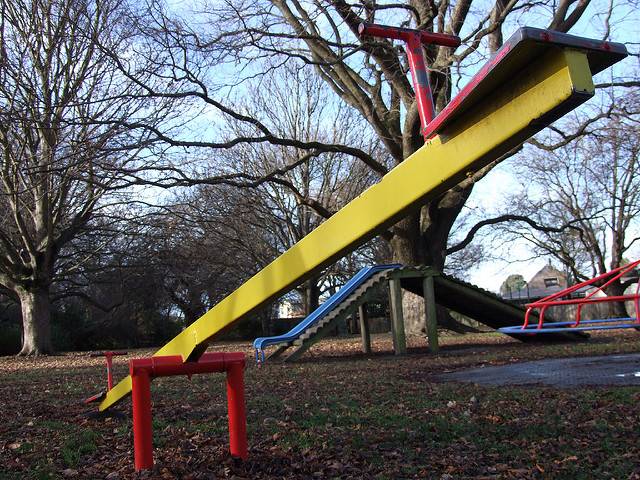# Substituting A Given Value For A Variable

Student View (Opens in new window)# Lesson Overview

Using a balance scale, students decide whether a certain value of a variable makes a given equation or inequality true. Then students extend what they learned using the balance scale to substituting a given value for a variable into an equation or inequality to decide if that value makes the equation or inequality true or false.

# Key Concepts

Students will extend what they know about substituting a value for a variable into an expression to evaluate that expression.

Equations and inequalities may contain variables. These equations or inequalities are neither true nor false. When a value is substituted for a variable, the equation or inequality then becomes true or false. If the equation or inequality is true for that value of the variable, that value is considered a solution to the equation or inequality.

# Goals and Learning Objectives

• Understand what solving an equation or inequality means.
• Use substitution to determine whether a given number makes an equation or inequality true.